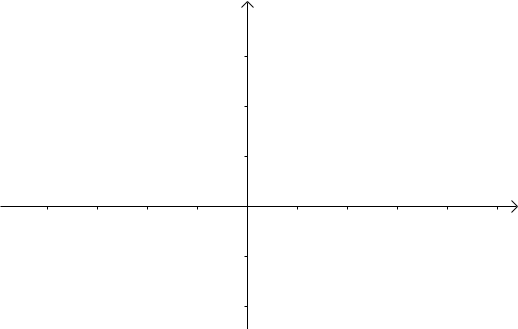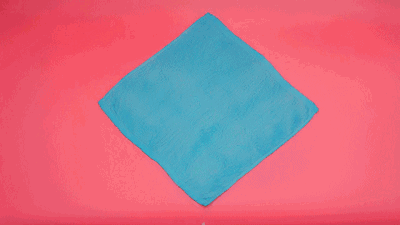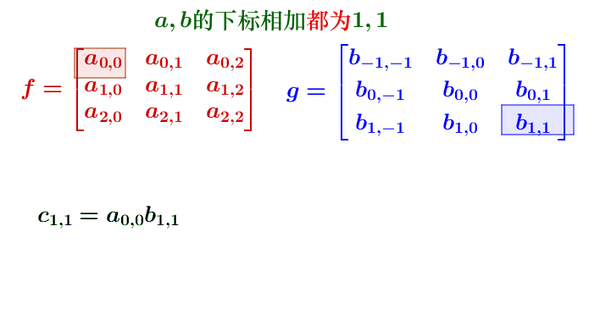# 0.做一个实验

## scipy.signal中

from scipy.signal import convolve2d as conv2d
A = np.array([[1, 2, 1], [2, 1, 2], [2, 1, 1]]) # A 为输入特征
W = np.array([[1, 1], [1, 2]])  # W 为卷积核
out1 = conv2d(A,W,mode='valid')
print(out1)


[[7 8]
[8 6]]

## tensorFlow中

import tensorflow as tf
a = tf.constant([1,2,1,2,1,2,2,1,1], dtype = tf.float32, shape = [1,3,3,1])
b = tf.constant([1,1,1,2], dtype = tf.float32,shape = [2,2,1,1])
with tf.Session() as sess:
print(sess.run(a))
print(b)
print(sess.run(c))


[[[[7.]
[8.]]

[[7.]
[6.]]]]

## 1.卷积的定义

Definition:
The convolution of $f$,and $g$ is tritten $f\ * g$,using an asterisk or star. It is defined as the intergral of the product of the two functions after one is reversed and shifted. As such, it is a particular kind of intergral transform:
$(f*g)(t)=\int_{-\infty}^{+\infty}f(\tau)g(t-\tau)$
While the symbol $t$ is used above, it need not represent the time domain. But in that context, the convolution formula can be described as a weighted average of the function $f(\tau)$ at the moment $t$ where the weighting is given by $g(-\tau)$ simply shifted by amount $t$. As $t$ changes,the weighting function emphasizes different parts of the input function.

For functions$f$,$g$ supported on only$[0,\infty)$(i.e, zero for negative argumenets), the integration limits can be truncated, resulting in:
$(f*g)(t)=\int_0^tf(\tau)g(t-\tau)d\tau \ for f,g:[0,\infty)\rightarrow \mathbb R$

$(f*g)(t)=\sum_{-\infty}^{+\infty}f(\tau)g(t-\tau)$

$42=(2\times10^0+4\times10^1)$可看作序列(2,4)
$137=(7\times10^0+3\times10^1+1\times10^2)$可看作序列（7,3,1）

$x$ 0 1
$f(x)$ 2 4
$y$ 0 1 2
$f(x)$ 7 3 1

1. $x+y=0$的情况：$(x=0,y=0)$
2. $x+y=1$的情况：$(x=0,y=1)$,$(x=1,y=0)$
3. $x+y=2$的情况：$(x=0,y=2)$,$(x=1,y=1)$
4. $x+y=3$的情况：$(x=1,y=2)$
(2,4)卷积(7,1,3) = (2X7, 2X3+4X7, 2X1+4X3, 4X1) = (14,34,14,4)
而14+34X10+14X100+4X1000 = 42X137
可以看出，一个卷积操作，要把所有的$t$遍历到（动态来看，就是一条滑动的直线）。对于每个$t$$x+y=t$都为一条斜对角直线，这条直线上所有的$(x,y)$序列所表示的$f(x)$$g(y)$,都要做乘法操作，然后求和。执行这样的操作一直到这条直线滑动完毕，就完成了一个卷积操作。其他人介绍卷积时，会添加一个生动的卷毛巾的动图，可以搜索看一下，加深理解程度。# 矩阵的卷积到底要不要翻转That is a problem!!，不过，最终，我找到了答案。

1.上文可以清晰地看到数学中卷积运算的特点：

2.卷积神经网络的卷积本质上是一种spatial filter 为什么这么说呢？

a）平滑滤波
b）边缘提取

3.此“卷积”与彼“卷积”的联系与区别 ：
**最直观的就是：是否进行翻转，然后再进行对应元素的加权求和。 **

• 数学中卷积，主要是为了诸如信号处理、求两个随机变量和的分布等而定义的运算，所以需要“翻转”是根据问题的需要而确定的
• 卷积神经网络中“卷积”，是为了提取图像的特征，其实只借鉴了“加权求和”的特点
• 还有一点一定要说的是：数学中的“卷积核”都是已知的或者给定的，卷积神经网络中“卷积核”本来就是trainable的参数，不是给定的，根据数据训练学习的，那么翻不翻转还有什么关系呢？因为无论翻转与否对应的都是未知参数！

04-098454

#### 求卷积层对卷积核导数旋转180的解释10-083791

#### 形象理解卷积（卷积运算为何要翻转）

04-252673

#### 卷积核翻转方法

05-165万+

#### 理解图像卷积操作的意义

07-19222

#### 深度学习面试题15：卷积核需要旋转180度

07-038万+

#### 深入理解卷积层，全连接层的作用意义

03-181万+

#### CNN误差反传时旋转卷积核的简明分析

11-195万+

#### 了解这些后，再去决定要不要买mac

02-293万+

#### CNN 中， 1X1卷积核到底有什么作用呢？

07-182万+

#### 一文读懂卷积神经网络中的1x1卷积核

05-23663

#### CV Code|计算机视觉开源周报20200503期

09-074541

#### 深度理解卷积--使用numpy实现卷积

07-312万+

#### 矩阵卷积运算的具体过程，很简单

02-02298

#### 为什么CNN中的卷积核要旋转180度？

11-141189

#### 深入理解反卷积

10-215万+

#### 卷积神经网络CNN（1）——图像卷积与反卷积（后卷积，转置卷积）

01-1719万+

#### C/C++学习指南全套教程

09-11©️2020 CSDN 皮肤主题: 大白 设计师: CSDN官方博客点击重新获取扫码支付1.余额是钱包充值的虚拟货币，按照1:1的比例进行支付金额的抵扣。
2.余额无法直接购买下载，可以购买VIP、C币套餐、付费专栏及课程。余额充值